### Home > CALC > Chapter 1 > Lesson 1.2.1 > Problem1-24

1-24.
1. Sketch the graph of the piecewise function g(x) below. 1-24 HW eTool (Desmos). Homework Help ✎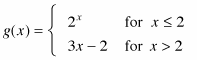1. State the domain and range of g(x).

2. Is g(x) continuous at x = 2? Explain.

3. Is g(x) continuous on all values of x?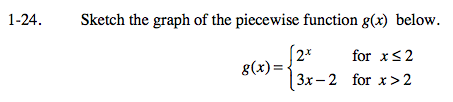Click on the circles next to each equation in the eTool below to view it.
Click the link at right for the full version of the eTool: Calc 1-24 HW eTool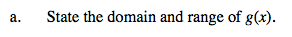Consider the domain and range of each piece separately . Then combine.
Domain: Both 2x and 3x − 2 have a domain of all real numbers.
Range: 2x on x ≤ 2 has a range of 0 < y ≤ 4 and 3x − 2 on x > 2 has a range of 4 < y.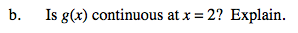Later in this course we will learn a formal definition of continuity. For now, think about what continuous means to you. Now look at the graph at x = 2. Make a conclusion and justify.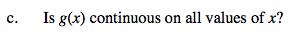Consider other values of x, other than x = 2. Is the function continuous at these point? Is there anywhere where the function will not be continuous?